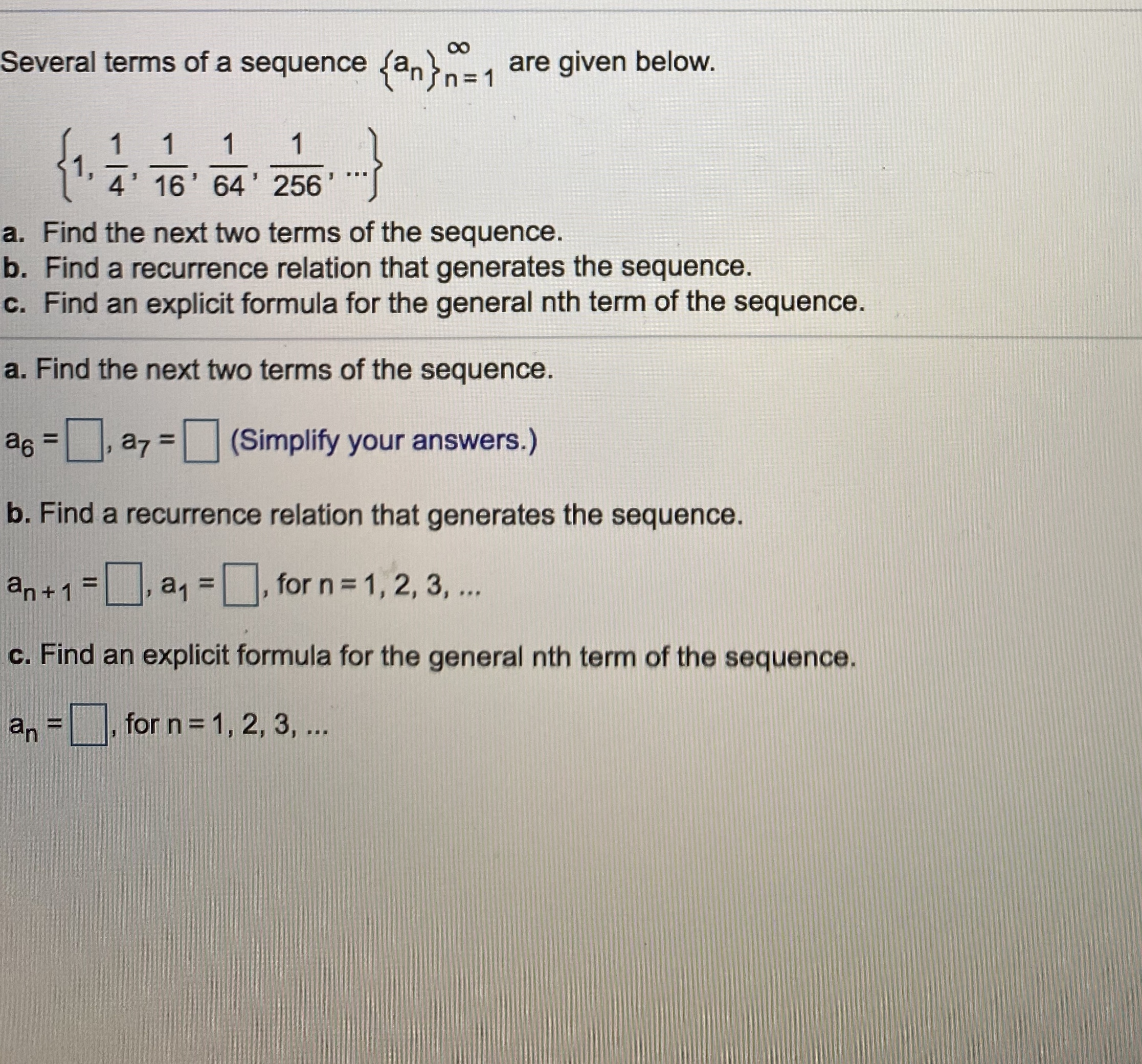# 00 Several terms of a sequence (an}n31 are given below. {1. 1 1 1 4' 16' 64' 256 a. Find the next two terms of the sequence. b. Find a recurrence relation that generates the sequence. c. Find an explicit formula for the general nth term of the sequence. a. Find the next two terms of the sequence. as =, a7 = (Simplify your answers.) %3D %3D b. Find a recurrence relation that generates the sequence. an+1 = a, =, for n = 1, 2, 3, .. %3D c. Find an explicit formula for the general nth term of the sequence. an = %3D for n = 1, 2, 3, ...

Question

3help_outlineImage Transcriptionclose00 Several terms of a sequence (an}n31 are given below. {1. 1 1 1 4' 16' 64' 256 a. Find the next two terms of the sequence. b. Find a recurrence relation that generates the sequence. c. Find an explicit formula for the general nth term of the sequence. a. Find the next two terms of the sequence. as =, a7 = (Simplify your answers.) %3D %3D b. Find a recurrence relation that generates the sequence. an+1 = a, =, for n = 1, 2, 3, .. %3D c. Find an explicit formula for the general nth term of the sequence. an = %3D for n = 1, 2, 3, ... fullscreen

### Want to see this answer and more?

Experts are waiting 24/7 to provide step-by-step solutions in as fast as 30 minutes!*

*Response times may vary by subject and question complexity. Median response time is 34 minutes for paid subscribers and may be longer for promotional offers.
Tagged in
Math
Calculus

### Other